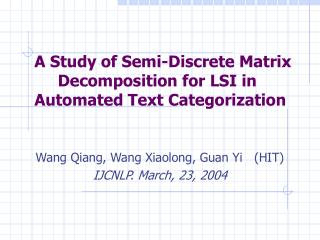DownloadDownload PresentationA Study of Semi-Discrete Matrix Decomposition for LSI in Automated Text Categorization

# A Study of Semi-Discrete Matrix Decomposition for LSI in Automated Text Categorization

Télécharger la présentation## A Study of Semi-Discrete Matrix Decomposition for LSI in Automated Text Categorization

- - - - - - - - - - - - - - - - - - - - - - - - - - - E N D - - - - - - - - - - - - - - - - - - - - - - - - - - -
##### Presentation Transcript

1. A Study of Semi-Discrete Matrix Decomposition for LSI in Automated Text Categorization Wang Qiang, Wang Xiaolong, Guan Yi (HIT) IJCNLP. March, 23, 2004

2. Plan of talk • A representation of a new text categorization technique based on: • Latent Semantic Indexing (1988. S.T.Dumais LSI-SDD) • LSI + kNN algorithm • Comparative evaluation of the new technique wrt previous work • kNN algorithm Solely (Duda and Hart, Pattern Classification and Scene analysis, 1973)

3. Text categorization • A fundamental problem of splitting a large text corpus into a number of semantic categories (predefined). • Definition: A: test documents B: semantic categories f : model • The problem has many real-world applications. • Search engines. • Information push.

4. Feature re-parameterisation • Latent Semantic Indexing (LSI) • attempts to solve the synonyms and polysemous problem • LSI differs from previous attempts at using reduced-space models : • LSI is able to represent and manipulate large data sets, making it viable for real-world applications • both terms and documents are explicitly represented in the same space • Each dimension is merely assumed to represent one or more semantic relationships in the term-document space

5. Feature re-parameterisation • Application of LSI • LSI via the singular value decomposition (SVD) • The most common method to LSI • Orthogonal matrix • Require more storage than the original matrix • LSI via the semi-discrete matrix decomposition (SDD) • it typically provides a more accurate approximation for far less storage

6. Feature re-parameterisation Singular Value Decomposition (SVD) • Approximating the Term-Document Matrix • Term-Document matrix A (m × n) , r=rank (A) • The SVD decomposes A: where U (m × r) ,V (r × n), Σ (r × r) • The truncated SVD : (k«r) where Uk and Vk consist of the first k columns of U and V respectively, and Σk is the leading k × k principal submatrix of Σ

7. Feature re-parameterisation Singular Value Decomposition (SVD) • Analysis n k n k k m = (a) (b)

8. Feature re-parameterisation Semi-Discrete Matrix Decomposition (SDD) • Approximating the Term-Document Matrix • Term-Document matrix A (m × n) , r=rank (A) • The SDD decomposes A : where U (m × r) ,V (r × n), Σ (r × r) • The truncated SDD : • different from SVD: Uk,Vk, which entries constrained to be in the set S = {-1, 0, 1}.

9. Feature re-parameterisation Semi-Discrete Matrix Decomposition (SDD) • SDD method s.t. • Comparison with SVD on storage

10. Feature re-parameterisation Semi-Discrete Matrix Decomposition (SDD) • Query processing （Test document） • We can process queries using approximation for A : (α=0) • Similarity

11. Classifier Algorithm k Nearest Neighbor (kNN) • LSI + kNN algorithm • Index the training set • Use SDD to map each document vector into a lower dimensional space. • For each document to be classified, retrieve its k most similar documents from the training set .Call this set . • For each category C, compute its relevance as: Where is the subset of documents in that are relevant to C

12. Classifier Algorithm k Nearest Neighbor (kNN) • Multi-class kNN • SCut • assigns to each category a threshold t(C) • assign a document to category C if ≥ t(C). • Loo (Leave-one-out) Cross-Validation • For each document d in the training set, use every other document in the training set to assign scores • set the values of t(C) to be those which produce optimal performance over this set of scores

13. Comparative evaluation Experiment • Data Sets and Protocol • Category criteria – Chinese Library Classification • Date sets: train sets (9,115) ,test sets (1,742) • Feature selection (5,362)- Expected Cross Entropy (ECE) • term-weighting formula

14. Comparative evaluation Experiment • Data Sets and Protocol • the value of k (kNN) is set to 50 through m-way cross-validation. • the values of t(C) for the SCut method is set through Leave-one-out Cross-validation Algorithm • Rank-k approximation in SDD (140 is optimal).

15. Comparative evaluation Experiment • Evaluation • the effectiveness measures of precision, recall and F1 are defined respectively .

16. Comparative evaluation Experiment • Results • Efficient comparison: • Precision,recall,F1 (macro-)

17. Comparative evaluation Experiment 36% 1.4% 9.48%

18. Comparative evaluation Experiment • The macro-averaged F1 score curves for each category using k-NN VSM versus k-NN LSI

19. Conclusion • LSI (SDD) is a promising technique for text categorization. • Comparison to SVD ( including VSM), SDD can achieve: • similar or higher performance • Much lower storage cost • Little executive time • LSI (SDD) is a technique that warrants further investigation for text categorization.

20. Acknowledgements Thank you all! Wang Qiang http://www.insun.hit.edu.cn E-mail:qwang@insun.hit.edu.cn